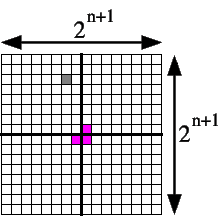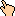## 1996-09 SolutionA proof by induction:

• Base Case #1: n = 0 means a 1x1 chessboard. Removing that one tile leaves nothing left to be tiled, so we've tiled the chessboard with 0 tiles.
• Base Case #2: n = 1 means a 2x2 chessboard. Removing that one tile leaves exactly a triomino, which we put down at the proper orientation.
• Induction step: We assume we can tile a 2n by 2n chessboard with a square removed from it. To tile the 2n+1 by 2n+1 chessboard with a square removed from it, we divide the chessboard down the middle horizontally and vertically. This cuts the chessboard into 4 equal 2n by 2n blocks. The missing square will be in one of those sections, WLOG let's say it's in the upper-left section. We know from induction that we can tile that section. The remaining three sections are tiled by removing the square closest to the center from each of these 2n by 2n sections. These 3 sections with that square removed can be tiled by induction. The central three squares removed (shown in magenta below) perfectly form a triomino, so we use one additional triomino to fill that region, and we're done.

WWW Maven: Dan Garcia (ddgarcia@cs.berkeley.edu)Send me feedback